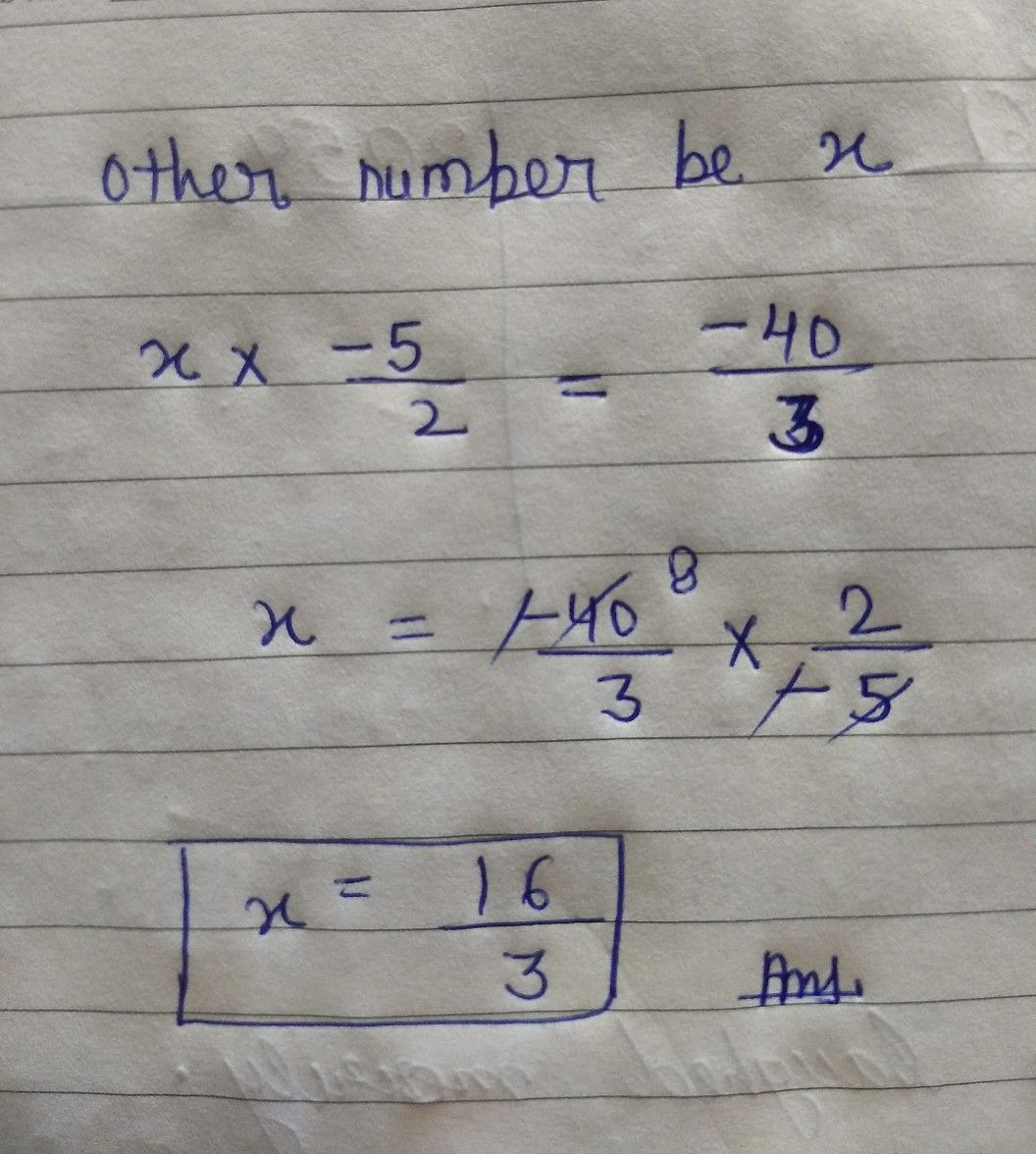Symbol
Problem$Q8.$ The product of two rational number $15$ $403$ $403$ If one of the number is $52,$ find the other. $O9.$ Evaluate $\left(6$ $.1+8n.1$ $+3n1+2n-1\right)^{n}.1$